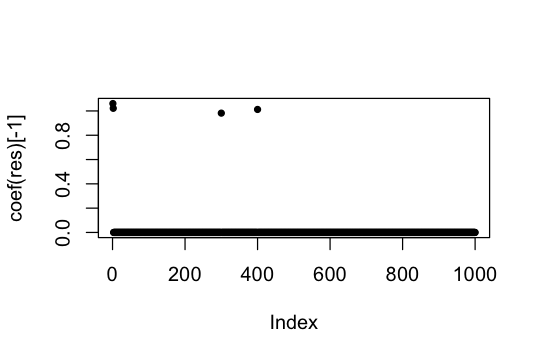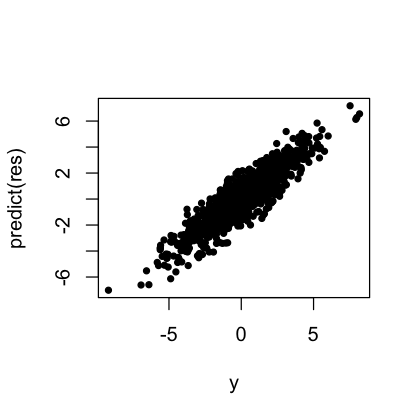A minimal example

2021-11-12

In this short vignette, we fit a sparse linear regression model with up to $$L > 0$$ non-zero effects. Generally, there is no harm in over-stating $$L$$ (that is, the method is pretty robust to overfitting), except that computation will grow as $$L$$ grows.

Here is a minimal example:

library(susieR)
set.seed(1)
n    <- 1000
p    <- 1000
beta <- rep(0,p)
beta[c(1,2,300,400)] <- 1
X   <- matrix(rnorm(n*p),nrow=n,ncol=p)
y   <- X %*% beta + rnorm(n)
res <- susie(X,y,L=10)
plot(coef(res)[-1],pch = 20)Plot the ground-truth outcomes vs. the predicted outcomes:

plot(y,predict(res),pch = 20)